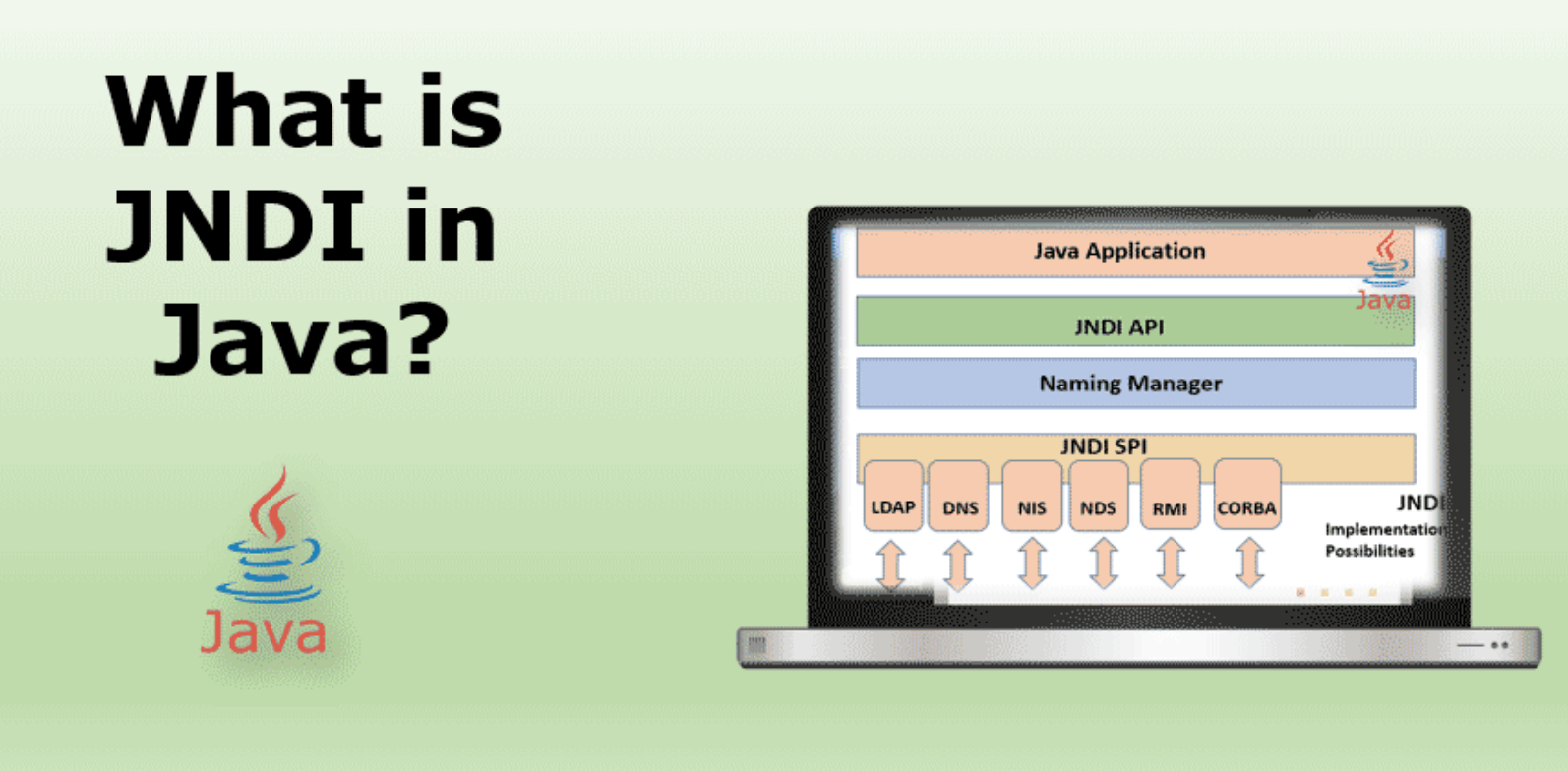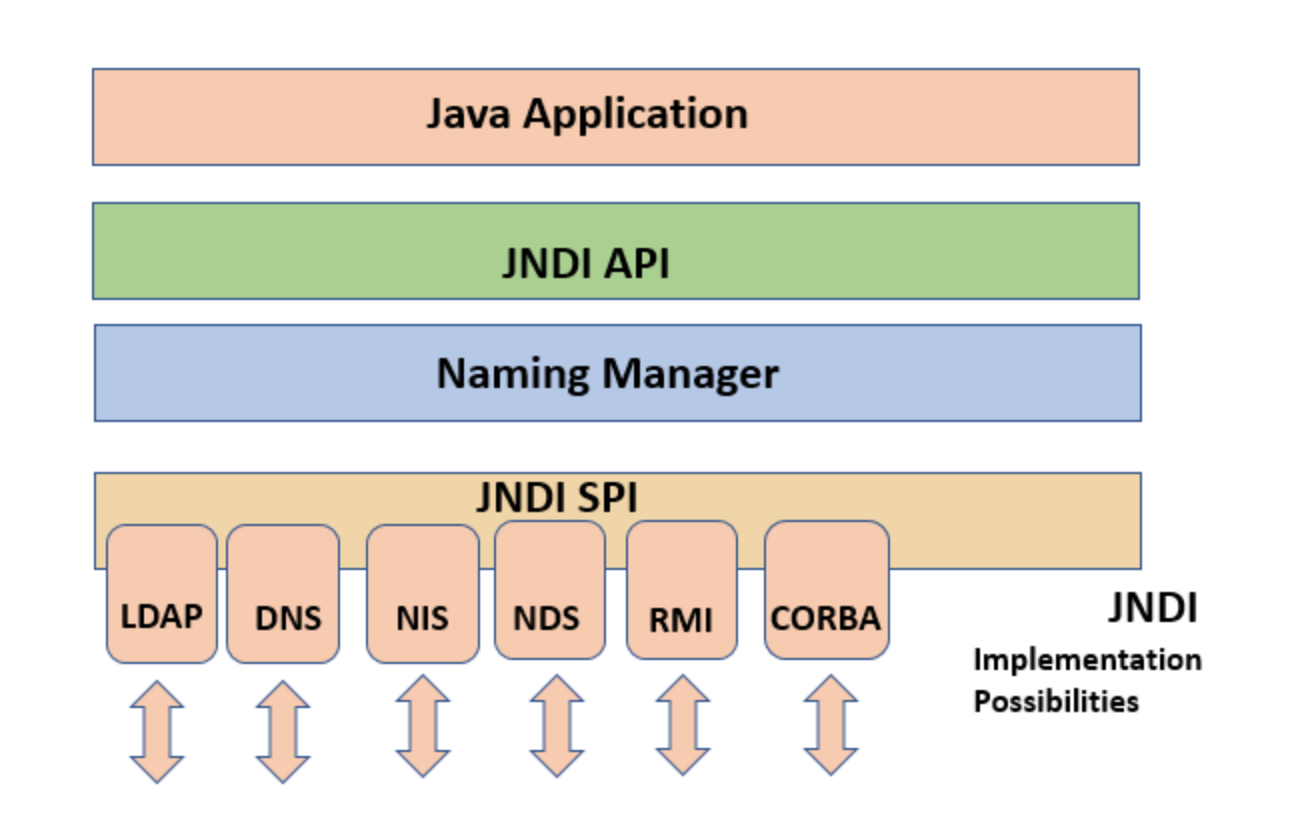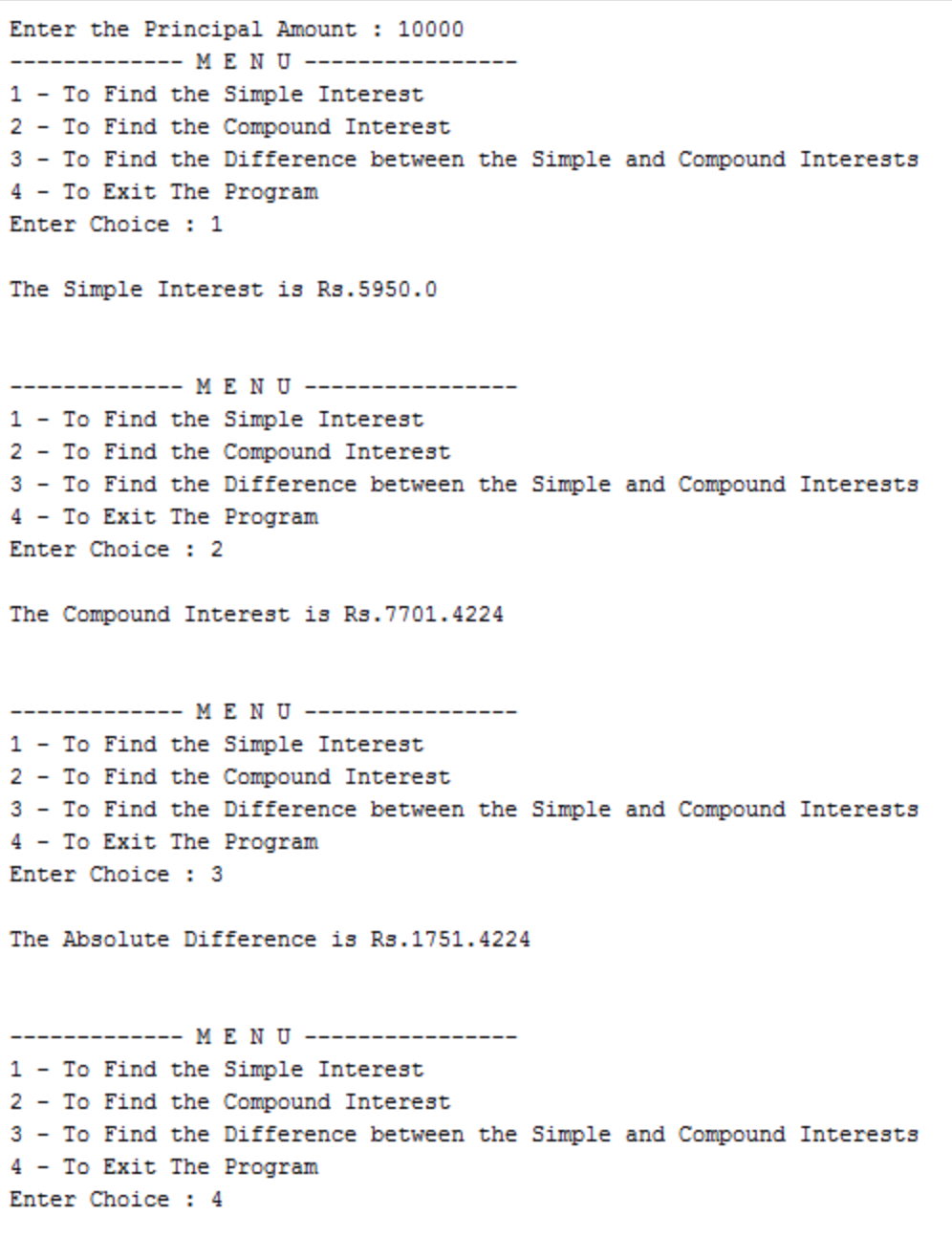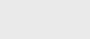2年前 (2021-12-17) |   抢沙发  412

[收起] 文章目录Java命名和目录接口是Java编程语言中接口的名称（JNDI）。它是一个API（应用程序接口），与服务器一起工作，可以使用命名约定从数据库获取文件。命名约定可以是单个短语或单词。还可以将其合并到套接字中，使用服务器在项目中传输数据文件或平面文件来实现套接字socket编程。它也可以在浏览器中的网页中使用，其中有许多目录的实例。JNDI为Java用户提供了使用Java编码语言在Java中搜索对象的工具。

## Java中JNDI的体系结构• 轻量级目录访问协议
• DNS-域名服务
• RMI-Java远程方法调用

## JNDI包

Java中有五个使用JNDI SPI的包。其中一些包是`javax.naming``javax.naming`是一个包，其中包含用于访问命名服务的类和接口。有查找、列表绑定、名称等功能。第二个是`java.naming.directory`这个包有助于将数据作为对象获取，是java的高级版本`java.naming directory`。还有其他`java. naming. event``java. naming. spi`

• JDBC（Java数据库连接）
• JMS（Java消息传递服务）
• EJB（企业Java bean）

JDBC用于数据库处理，JMS是消息传递服务应用程序。EJB与用于运行Java程序的Netbeans和Eclipse平台一起运行。这些软件包与使用它们的技术一起提供。

JNDI还与LDAP服务提供程序一起使用。有一系列代码用Java语言运行编程应用程序。

Java编程语言中有一个`bind（）``look up（）`，用于命名对象和从目录中查找对象。

``Context.bind(“name”, object)``

``Object hello= Context.lookup(“name”)``

JNDI及其应用程序在数据分析行业中得到了广泛的应用，在数据分析行业中，有大量数据需要挖掘，并且数据的某些方面存储在不同的目录中，文件存储在不同的文件夹中。它在电信行业中有着广泛的应用，在电信行业中，人们根据每小时的通话率来计算账单。

## Java中的JNDI示例

``````import java.io.*;
class Assignment1
{
public static void main(String[] args) throws Exception
{
System.out.print("Enter the Principal Amount : ");//prompt for entering the principal amount
float P = Float.parseFloat(ob.readLine());//accepting the principal amount
int choice = 0;
do{
choice = 0;// reseting the user's choice
System.out.println("------------- M E N U ----------------");
System.out.println("1 - To Find the Simple Interest");
System.out.println("2 - To Find the Compound Interest");
System.out.println("3 - To Find the Difference between the Simple and Compound Interests");
System.out.println("4 - To Exit The Program");
System.out.print("Enter Choice : ");//prompting for user's choice
System.out.println("");// line feed between menu and result
switch(choice)
{
case 1://for simple interest
System.out.println("The Simple Interest is Rs."+simple(P));
break;
case 2://for compound interset
System.out.println("The Compound Interest is Rs."+compound(P));
break;
case 3://for difference between simple and compound interests
System.out.println("The Absolute Difference is Rs."+(compound(P)-simple(P)));
break;
case 4:
System.out.println("Program Terminated");
break;
default://for a wrong choice entered by the user
System.out.println("Invalid Option");
}//end of switch(choice)
System.out.println("\n");//linefeed between two consecutive choices by the user
}while(choice!=4);//end of do-while
}//end of main
public static float simple(float p)//to calculate the simple interest
{
return (float)((p*8.5*7.0)/100.0); //returning the simple interest
}//end of simple
public static float compound(float p)//to calculate the compound interest
{
return (p*(float)(Math.pow((1.0+(8.5/100.0)),7.0)-1.0));//returning the compound interest
}//end of compound
}//end of class``````## 总结### 注册